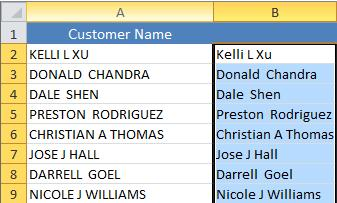# How To Use VBA Convert to Proper Case In Excel

Oct 24, 2020 • edited Oct 25, 2020Below we will look at a program in Excel VBA that converts text to proper case. That is, the first letter in each word in uppercase, and all other letters in lowercase.

Situation:1. First, we declare two Range objects. We call the Range objects rng and cell.

``````          Dim rng As Range, cell As Range
``````

2. We initialize the Range object rng with the selected range.

``````          Set rng = Selection
``````

3. We want to check each cell in a randomly selected range (this range can be of any size). In Excel VBA, you can use the For Each Next loop for this. Add the following code lines:

``````          For Each cell In rng
``````

Next cell

Note: rng and cell are randomly chosen here, you can use any names. Remember to refer to these names in the rest of your code.

4. To ignore a cell that contains a formula, add the following code line between For Each and Next (only if cell.HasFormula is false we continue).

``````          If Not cell.HasFormula Then
``````

End If

5. Next, we want to convert each word in this range to ‘proper case’. You can use the worksheet function Proper for this task. Add the following code line in your if statement.

``````          cell.Value = WorksheetFunction.Proper(cell.Value)
``````

6. Test the program.

Result:#Tutorial#How To#VBA#String Manipulation

How To Use VBA Controls Collection In Excel

How To Use VBA Dynamic Range In Excel